Related Articles
Types Of Activation Function in ANN
• Difficulty Level : Medium
• Last Updated : 22 Jan, 2021

The biological neural network has been modeled in the form of Artificial Neural Networks with artificial neurons simulating the function of a biological neuron. The artificial neuron is depicted in the below picture: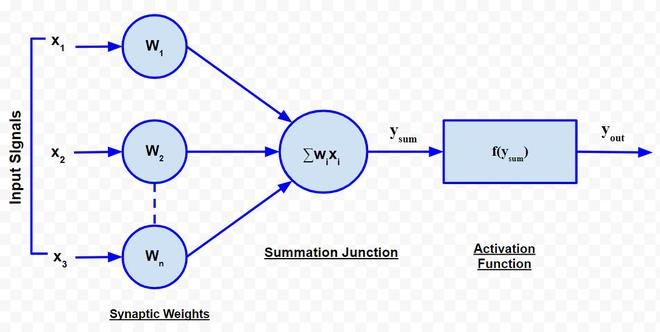Structure of an Artificial Neuron

Each neuron consists of three major components:

1. A set of ‘i’ synapses having weight wi. A signal xi forms the input to the i-th synapse having weight wi. The value of any weight may be positive or negative. A positive weight has an extraordinary effect, while a negative weight has an inhibitory effect on the output of the summation junction.
2. A summation junction for the input signals is weighted by the respective synaptic weight. Because it is a linear combiner or adder of the weighted input signals, the output of the summation junction can be expressed as follows:3. A threshold activation function (or simply the activation function, also known as squashing function) results in an output signal only when an input signal exceeding a specific threshold value comes as an input. It is similar in behaviour to the biological neuron which transmits the signal only when the total input signal meets the firing threshold.

Types of Activation Function :

There are different types of activation functions. The most commonly used activation function are listed below:

A. Identity Function: Identity function is used as an activation function for the input layer. It is a linear function having the formAs obvious, the output remains the same as the input.

B. Threshold/step Function: It is a commonly used activation function. As depicted in the diagram, it gives 1 as output of the input is either 0 or positive. If the input is negative, it gives 0 as output. Expressing it mathematically,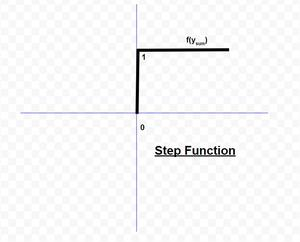The threshold function is almost like the step function, with the only difference being a fact thatis used as a threshold value instead of . Expressing mathematically,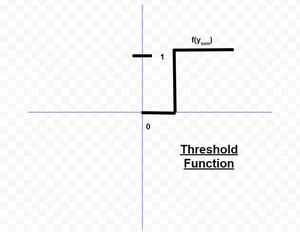C. ReLU (Rectified Linear Unit) Function: It is the most popularly used activation function in the areas of convolutional neural networks and deep learning. It is of the form: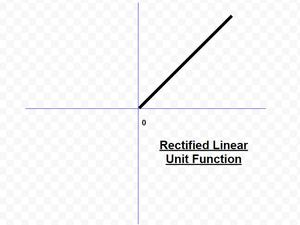This means that f(x) is zero when x is less than zero and f(x) is equal to x when x is above or equal to zero. This function is differentiable, except at a single point x = 0. In that sense, the derivative of a ReLU is actually a sub-derivative.

D. Sigmoid Function: It is by far the most commonly used activation function in neural networks. The need for sigmoid function stems from the fact that many learning algorithms require the activation function to be differentiable and hence continuous. There are two types of sigmoid function:

1. Binary Sigmoid Function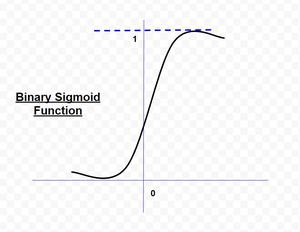A binary sigmoid function is of the form:, where k = steepness or slope parameter, By varying the value of k, sigmoid function with different slopes can be obtained. It has a range of (0,1).  The slope of origin is k/4. As the value of k becomes very large, the sigmoid function becomes a threshold function.

2. Bipolar Sigmoid Function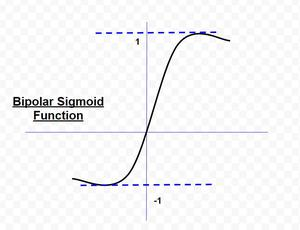A bipolar sigmoid function is of the formThe range of values of sigmoid functions can be varied depending on the application. However, the range of (-1,+1) is most commonly adopted.

E. Hyperbolic Tangent Function: It is bipolar in nature. It is a widely adopted activation function for a special type of neural network known as Backpropagation Network. The hyperbolic tangent function is of the formThis function is similar to the bipolar sigmoid function.My Personal Notes arrow_drop_up